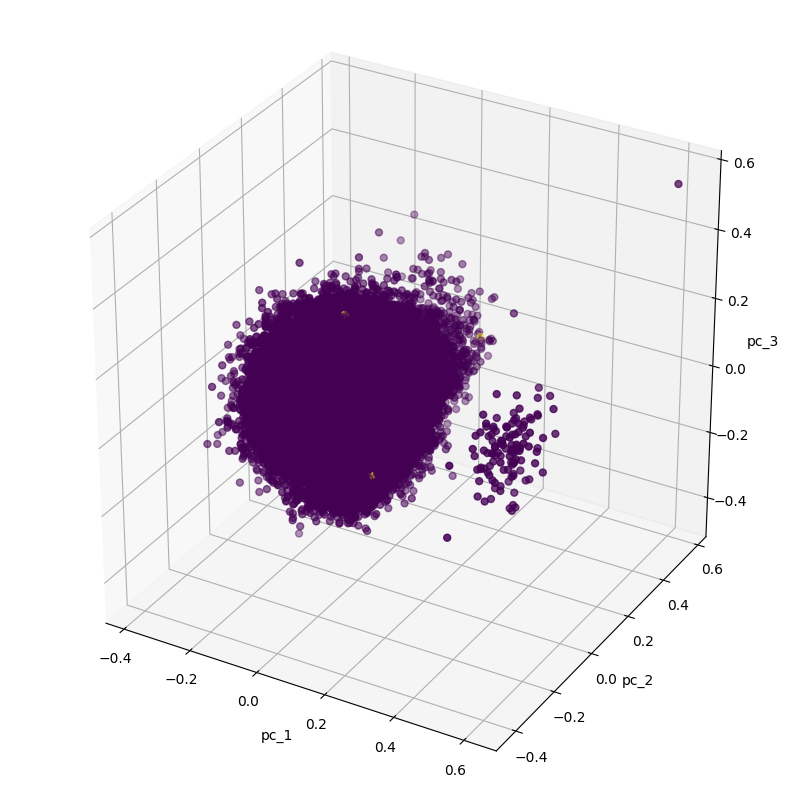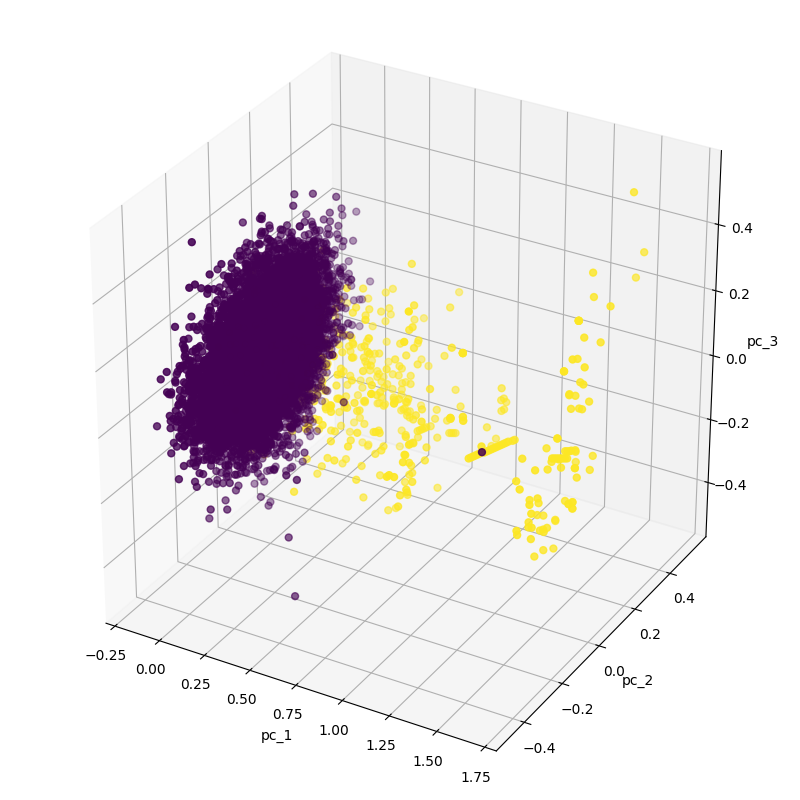1595578200

# Applying Anomaly Detection with Autoencoders to Fraud Detection

I recently read an article called Anomaly Detection with Autoencoders. The article was based on generated data, so it sounded like a good idea to apply this idea to a real-world fraud detection task and validate it.

I decided to use Credit Card Fraud Dataset From Kaggle*:

The datasets contains transactions made by credit cards in September 2013 by european cardholders.

This dataset presents transactions that occurred in two days, where we have 492 frauds out of 284,807 transactions. The dataset is highly unbalanced, the positive class (frauds) account for 0.172% of all transactions.

It is a very unbalanced dataset and a good candidate to identify fraud through anomalies.

We are going to do a smaller plot after decreasing our dimensions from 30 to 3 with Principal Component Analysis. This data has 32 columns where the first column is the time index, 29 unknown features, 1 transaction amount, and 1 class. I will ignore the time index since it is not stationary.

``````def show_pca_df(df):
x = df[df.columns[1:30]].to_numpy()
y = df[df.columns].to_numpy()

x = preprocessing.MinMaxScaler().fit_transform(x)
pca = decomposition.PCA(n_components=3)
pca_result = pca.fit_transform(x)
print(pca.explained_variance_ratio_)

pca_df = pd.DataFrame(data=pca_result, columns=['pc_1', 'pc_2', 'pc_3'])
pca_df = pd.concat([pca_df, pd.DataFrame({'label': y})], axis=1)

ax = Axes3D(plt.figure(figsize=(8, 8)))
ax.scatter(xs=pca_df['pc_1'], ys=pca_df['pc_2'], zs=pca_df['pc_3'], c=pca_df['label'], s=25)
ax.set_xlabel("pc_1")
ax.set_ylabel("pc_2")
ax.set_zlabel("pc_3")
plt.show()

show_pca_df(df)
view raw
anomaly_detection_part1_1.py hosted with ❤ by GitHub
``````Your first reaction could be that there are two clusters and this would be an easy task but fraud data is yellow points! There are three visible yellow points in the large cluster. So let’s subsample the normal data while keeping the number of fraud data.

``````df_anomaly = df[df[df.columns] > 0]
df_normal = df[df[df.columns] == 0].sample(n=df_anomaly.size, random_state=1, axis='index')
df = pd.concat([ df_anomaly, df_normal])

show_pca_df(df)
view raw
anomaly_detection_part1_2.py hosted with ❤ by GitHub
``````#keras #anomaly-detection #deep-learning #tensorflow #fraud-detection #deep learning

## Buddha Community1618128600

## Credit Card Fraud Detection via Machine Learning: A Case Study

This is the second and last part of my series which focuses on Anomaly Detection using Machine Learning. If you haven’t already, I recommend you read my first article here which will introduce you to Anomaly Detection and its applications in the business world.

In this article, I will take you through a case study focus on Credit Card Fraud Detection. It is important that credit card companies are able to recognize fraudulent credit card transactions so that customers are not charged for items that they did not purchase. So the main task is to identify fraudulent credit card transactions by using Machine learning. We are going to use a Python library called PyOD which is specifically developed for anomaly detection purposes.

#machine-learning #anomaly-detection #data-anomalies #detecting-data-anomalies #fraud-detection #fraud-detector #data-science #machine-learning-tutorials1595578200

## Applying Anomaly Detection with Autoencoders to Fraud Detection

I recently read an article called Anomaly Detection with Autoencoders. The article was based on generated data, so it sounded like a good idea to apply this idea to a real-world fraud detection task and validate it.

I decided to use Credit Card Fraud Dataset From Kaggle*:

The datasets contains transactions made by credit cards in September 2013 by european cardholders.

This dataset presents transactions that occurred in two days, where we have 492 frauds out of 284,807 transactions. The dataset is highly unbalanced, the positive class (frauds) account for 0.172% of all transactions.

It is a very unbalanced dataset and a good candidate to identify fraud through anomalies.

We are going to do a smaller plot after decreasing our dimensions from 30 to 3 with Principal Component Analysis. This data has 32 columns where the first column is the time index, 29 unknown features, 1 transaction amount, and 1 class. I will ignore the time index since it is not stationary.

``````def show_pca_df(df):
x = df[df.columns[1:30]].to_numpy()
y = df[df.columns].to_numpy()

x = preprocessing.MinMaxScaler().fit_transform(x)
pca = decomposition.PCA(n_components=3)
pca_result = pca.fit_transform(x)
print(pca.explained_variance_ratio_)

pca_df = pd.DataFrame(data=pca_result, columns=['pc_1', 'pc_2', 'pc_3'])
pca_df = pd.concat([pca_df, pd.DataFrame({'label': y})], axis=1)

ax = Axes3D(plt.figure(figsize=(8, 8)))
ax.scatter(xs=pca_df['pc_1'], ys=pca_df['pc_2'], zs=pca_df['pc_3'], c=pca_df['label'], s=25)
ax.set_xlabel("pc_1")
ax.set_ylabel("pc_2")
ax.set_zlabel("pc_3")
plt.show()

show_pca_df(df)
view raw
anomaly_detection_part1_1.py hosted with ❤ by GitHub
``````Your first reaction could be that there are two clusters and this would be an easy task but fraud data is yellow points! There are three visible yellow points in the large cluster. So let’s subsample the normal data while keeping the number of fraud data.

``````df_anomaly = df[df[df.columns] > 0]
df_normal = df[df[df.columns] == 0].sample(n=df_anomaly.size, random_state=1, axis='index')
df = pd.concat([ df_anomaly, df_normal])

show_pca_df(df)
view raw
anomaly_detection_part1_2.py hosted with ❤ by GitHub
``````#keras #anomaly-detection #deep-learning #tensorflow #fraud-detection #deep learning1618310820

## These Tips Will Help You Step Up Anomaly Detection Using ML

In this article, you will learn a couple of Machine Learning-Based Approaches for Anomaly Detection and then show how to apply one of these approaches to solve a specific use case for anomaly detection (Credit Fraud detection) in part two.

A common need when you analyzing real-world data-sets is determining which data point stand out as being different from all other data points. Such data points are known as anomalies, and the goal of anomaly detection (also known as outlier detection) is to determine all such data points in a data-driven fashion. Anomalies can be caused by errors in the data but sometimes are indicative of a new, previously unknown, underlying process.

#machine-learning #machine-learning-algorithms #anomaly-detection #detecting-data-anomalies #data-anomalies #machine-learning-use-cases #artificial-intelligence #fraud-detection1604230740

## What is an Anomaly?

An anomaly by definition is something that deviates from what is standard, normal, or expected.

When dealing with datasets on a binary classification problem, we usually deal with a balanced dataset. This ensures that the model picks up the right features to learn. Now, what happens if you have very little data belonging to one class, and almost all data points belong to another class?

In such a case, we consider one classification to be the ‘normal’, and the sparse data points as a deviation from the ‘normal’ classification points.

For example, you lock your house every day twice, at 11 AM before going to the office and 10 PM before sleeping. In case a lock is opened at 2 AM, this would be considered abnormal behavior. Anomaly detection means predicting these instances and is used for Intrusion Detection, Fraud Detection, health monitoring, etc.

In this article, I show you how to use pycaret on a dataset for anomaly detection.

## What is PyCaret?

PyCaret is an open-source, low-code machine learning library in Python that aims to reduce the cycle time from hypothesis to insights. It is well suited for seasoned data scientists who want to increase the productivity of their ML experiments by using PyCaret in their workflows or for citizen data scientists and those **new to data science **with little or no background in coding. PyCaret allows you to go from preparing your data to deploying your model within seconds using your choice of notebook environment.

So, simply put, pycaret makes it super easy for you to visualize and train a model on your datasets within 3 lines of code!

So let’s dive in!

#anomaly-detection #machine-learning #anomaly #fraud-detection #pycaret1601280960

## Statistical techniques for anomaly detection

Anomaly and fraud detection is a multi-billion-dollar industry. According to a Nilson Report, the amount of global credit card fraud alone was USD 7.6 billion in 2010. In the UK fraudulent credit card transaction losses were estimated at more than USD 1 billion in 2018. To counter these kinds of financial losses a huge amount of resources are employed to identify frauds and anomalies in every single industry.

In data science, “Outlier”, “Anomaly” and “Fraud” are often synonymously used, but there are subtle differences. An “outliers’ generally refers to a data point that somehow stands out from the rest of the crowd. However, when this outlier is completely unexpected and unexplained, it becomes an anomaly. That is to say, all anomalies are outliers but not necessarily all outliers are anomalies. In this article, however, I am using these terms interchangeably.

There are numerous reasons why understanding and detecting outliers are important. As a data scientist when we make data preparation we take great care in understanding if there is any data point unexplained, which may have entered erroneously. Sometimes we filter completely legitimate outlier data points and remove them to ensure greater model performance.

There is also a huge industrial application of anomaly detection. Credit card fraud detection is the most cited one but in numerous other cases anomaly detection is an essential part of doing business such as detecting network intrusion, identifying instrument failure, detecting tumor cells etc.

A range of tools and techniques are used to detect outliers and anomalies, from simple statistical techniques to complex machine learning algorithms, depending on the complexity of data and sophistication needed. The purpose of this article is to summarise some simple yet powerful statistical techniques that can be readily used for initial screening of outliers. While complex algorithms can be inevitable to use, sometimes simple techniques are more than enough to serve the purpose.

Below is a primer on five statistical techniques.

#anomaly-detection #machine-learning #outlier-detection #data-science #fraud-detection